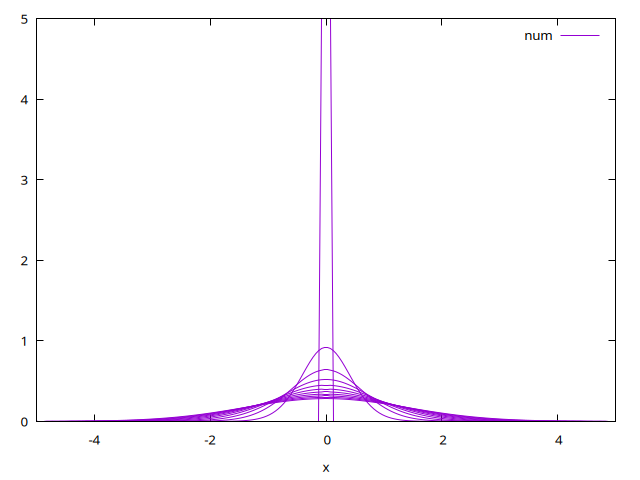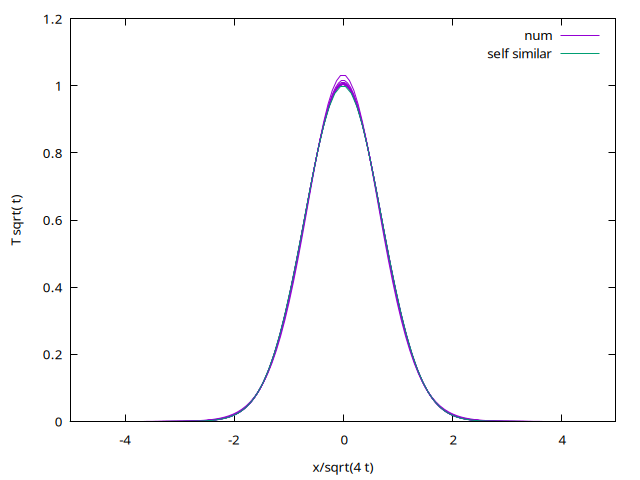# Resolution of heat equation

Resolution of heat equation \displaystyle \frac{\partial T}{\partial t}= \frac{\partial^2 T}{\partial x^2},\;\; \;\;\text{ with constant energy } \;\;\int_{-\infty}^{+\infty} T dx=1 with an implicit scheme.

#include "grid/cartesian1D.h"
#include "run.h"

scalar T[];
double dt;

int main() {
L0 = 10.;
X0 = -L0/2;
N = 200;
DT = .01 ;
#define epsilon 0.1
run();
}

initial temperature a “dirac”

event init (t = 0) {
foreach()
T[] =  1./epsilon*(fabs(x)<epsilon)/2;
boundary ({T});
}

print data

event printdata (t += 0.1; t < 1) {
foreach()
fprintf (stdout, "%g %g %g \n", x, T[], t);
fprintf (stdout, "\n \n");
}

integration

event integration (i++) {
double dt = DT;
scalar Told[] ;

finding the good next time step

  dt = dtnext (dt);

the explicit step \displaystyle \frac{ T -T_{old}}{dt} = \frac{ T(x+\Delta ) - 2 T(x) +T(x-\Delta )}{\Delta^2 } is written with Gauss Seidel trick (iteration n), it means that in the RHS we writte an implicit T(x): i.e. T^{n+1}(x) whereas T(x\pm \Delta) remains explicit i.e. T^{n}(x\pm\Delta) \displaystyle \frac{ T^{n+1} -T_{old}}{dt} = \frac{ T^n(x+\Delta ) - 2 T^{n+1}(x) +T^n(x-\Delta )}{\Delta^2 } so the new T^{n+1} : \displaystyle T^{n+1} (1 + 2 dt/ \Delta^2) = T_{old} + dt/ \Delta^2( T^n(x+\Delta ) +T^n(x-\Delta)) to speed up, one can do a relaxation \displaystyle T^{n+1} = (1-\omega) T^n + \omega ( T_{old} + dt/ \Delta^2( T^n(x+\Delta ) +T^n(x-\Delta)))/(1 + 2 dt/ \Delta^2) a loop is done until convergence T^{n+1} \simeq T^{n}

 foreach() Told[] = T[];
double eps=.0,Tn=0.,omega=.25;
do
{ eps=0;
foreach()
{
Tn = ( Told[] + (dt/sq(Delta))*(T[-1,0] + T[1,0])) / (1 + 2.*dt/sq(Delta));
eps = eps + sq(T[]-Tn);
T[] =   omega * Tn + (1-omega) * T[] ;
}
//  fprintf (stderr, "t=%g eps=%g \n",t, sqrt(eps));
}while(sqrt(eps)>1.e-12);
boundary ({T});
}

# Results

Then compile and run:

qcc  -g -O2 -DTRASH=1 -Wall -lm heat_imp.c -o heat
heat > out

or with make

 ln -s ../../Makefile Makefile
make heat_imp.tst;make heat_imp/plots
make heat_imp.c.html ; open heat_imp.c.html 

The solution as function of time is \displaystyle T = \frac{1}{2 \sqrt{\pi t} } e^{-x^2/(4 t)}

set output 'time.png'
set xlabel "x"
p[-5:5][:]'out'  t'num' w ltime evolution (script)

The self similar analytical solution is \theta = T \sqrt{t} function of \eta=x/\sqrt{4 t}: \displaystyle \theta = \frac{1}{2 \sqrt{\pi } } e^{-\eta^2}
set output 'selfsimilar.png'
set xlabel "x/sqrt(4 t)"
set ylabel "T sqrt( t)"
p[-5:5][:]'out'  u ($1/sqrt(4*$3)):($2*sqrt($3*pi)*2) t'num' w l,exp(-x*x) t'self similar'selfsimilar (script)

• Change DT to test the stablity